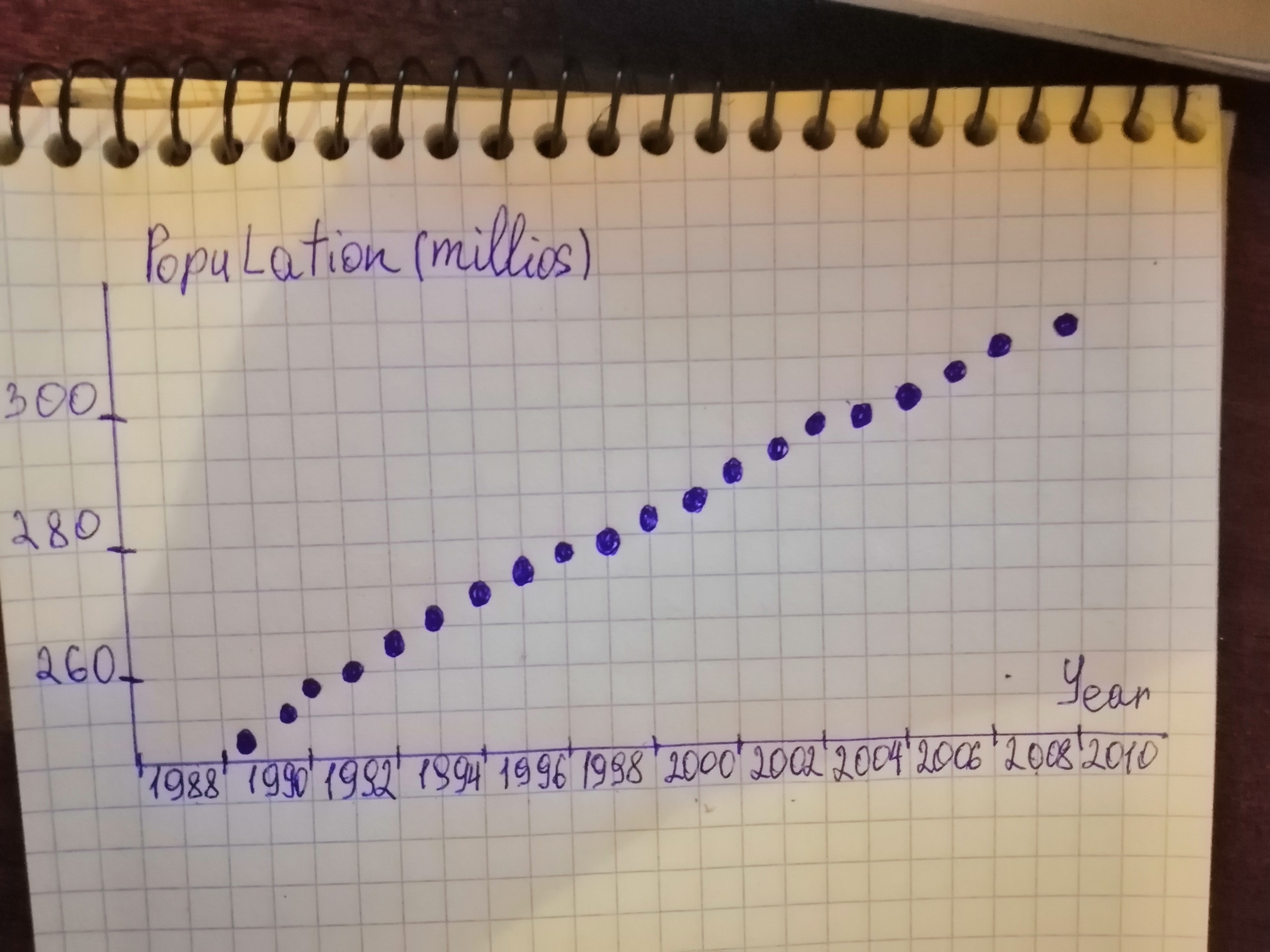# Question# The U.S. Census Bureau publishes information on the population of the United States in Current Population Reports.

Scatterplots
ANSWEREDThe U.S. Census Bureau publishes information on the population of the United States in Current Population Reports. The following table gives the resident U.S. population, in millions of persons, for the years 1990-2009. Forecast the U.S. population in the years 2010 and 2011

$$\begin{array}{|c|c|} \hline \text{Year} & \text{Population (millions)} \\ \hline 1990 & 250 \\ \hline 1991 & 253\\ \hline 1992 & 257\\ \hline 1993 & 260\\ \hline 1994 & 263\\ \hline 1995 & 266\\ \hline 1996 & 269\\ \hline 1997 & 273\\ \hline 1998 & 276\\ \hline 1999 & 279\\ \hline 2000 & 282\\ \hline 2001 & 285\\ \hline 2002 & 288\\ \hline 2003 & 290\\ \hline 2004 & 293\\ \hline 2005 & 296\\ \hline 2006 & 299\\ \hline 2007 & 302\\ \hline 2008 & 304\\ \hline 2009 & 307\\ \hline \end{array}$$

a) Obtain a scatterplot for the data.

b) Find and interpret the regression equation.

c) Mace the specified forecasts.2021-01-08

a) Year is on the horizontal axis and population is on the vertical axis.b) Let us first determine the necessary sums: $$\displaystyle\sum\ {x}_{{{i}}}={3990}$$
$$\displaystyle\sum\ {{x}_{{{i}}}^{{{2}}}}={79960670}$$
$$\displaystyle\sum\ {y}_{{{i}}}={5592}$$
$$\displaystyle\sum\ {x}_{{{i}}}\ {y}_{{{i}}}={11183199}$$ Next, we can determine $$\displaystyle{S}_{{x x}}$$ and $$\displaystyle{S}_{{{x}{y}}}$$
$$\displaystyle{S}_{{x x}}=\ \sum\ {{x}_{{{i}}}^{{{2}}}}\ -\ {\frac{{{\left(\sum\ {x}_{{{i}}}\right)}^{{{2}}}}}{{{n}}}}={79960670}\ -\ {\frac{{{3990}^{{{2}}}}}{{{20}}}}={665}$$
$$\displaystyle{S}_{{{x}{y}}}=\ \sum\ {x}_{{{i}}}{y}_{{{i}}}\ -\ {\frac{{{\left(\sum\ {x}_{{{i}}}\right)}{\left(\sum\ {y}_{{{i}}}\right)}}}{{{n}}}}={11183199}\ -\ {\frac{{{3990}\ \cdot\ {5592}}}{{{20}}}}={1995}$$ The estimate b of the slope $$\displaystyle\beta$$ is the ratio of $$\displaystyle{S}_{{{x}{y}}}$$ and $$\displaystyle{S}_{{x x}}$$: $$\displaystyle{b}=\ {\frac{{{S}_{{{x}{y}}}}}{{{S}_{{x x}}}}}=\ {\frac{{{1995}}}{{{665}}}}={3}$$ The mean is the sum of all values divided by the number of values: $$\displaystyle\overline{{{x}}}=\ {\frac{{\sum\ {x}_{{{i}}}}}{{{n}}}}=\ {\frac{{{6990}}}{{{20}}}}={1999.5}$$
$$\displaystyle\overline{{{y}}}=\ {\frac{{\sum\ {y}_{{{i}}}}}{{{n}}}}=\ {\frac{{{5592}}}{{{20}}}}={279.6}$$ The estimate a of the intercept $$\displaystyle\alpha$$ is the average of u decreased by the product of the estimate of the slope and the average of x. $$\displaystyle{a}=\ \overline{{{y}}}\ -\ {b}\overline{{{x}}}={279.6}\ -\ {3}\ \cdot\ {1999.5}=\ -{5718.9}$$ General least-squares equation: $$\displaystyle\hat{{{y}}}=\ \alpha\ +\ \beta\ {x}$$. Replace $$\displaystyle\alpha$$ by $$\displaystyle{a}=\ -{5505.3432}$$ and $$\displaystyle\beta$$ by $$\displaystyle{b}={2.8930}$$ in the general least-squares equation: $$\displaystyle{y}={a}\ +\ {b}{x}=\ -{5718.9}\ +\ {3}{x}$$

c) Let us evaluate the regression line of part (b) at $$\displaystyle{x}={2010}$$ and $$\displaystyle{x}={2011}$$. $$\displaystyle{y}=\ -{5718.9}\ +\ {3}{\left({2010}\right)}={311.1}$$
$$\displaystyle{y}=\ -{5718.9}\ +\ {3}{\left({2011}\right)}={314.1}$$ Thus the predicted U.S. population in 2010 is 311.1 million and predicted U.S. population in 2011 is 314.1 million.